# Top 28 Cheat Sheets for Machine Learning, Data Science

• 144
• 0 收藏，819 浏览

## Introduction

Data Science is an ever-growing field, there are numerous tools & techniques to remember. It is not possible for anyone to remember all the functions, operations and formulas of each concept. That’s why we have cheat sheets. But there are a plethora of cheat sheets available out there, choosing the right cheat sheet is a tough task. So, I decided to write this article.

Here I have selected the cheat sheets on the following criteria: comprehensiveness, clarity, and content.

After applying these filters, I have collated some 28 cheat sheets on machine learning, data science, probability, SQL and Big Data. For your convenience, I have segregated the cheat sheets separately for each of the above topics. There are cheat sheets on tools & techniques, various libraries & languages.

Read on to know which cheat sheet to use for a particular topic.

## Python for Data Science Cheat Sheets

### 1.Quick Guide to learn Python for Data Science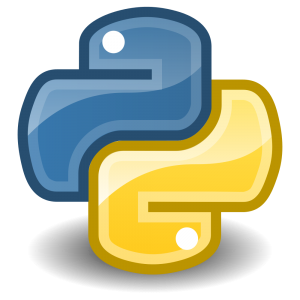If you are starting to learn Python, then this cheat sheet is the best resource for you. In this cheat sheet, you will find a step-by-step guide to learn Python. It gives out resources to follow, Python libraries you must know and few helpful tips.

### 2. Python for Data Science Cheat sheet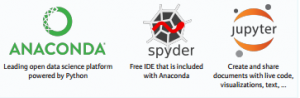This cheat sheet by Datacamp covers all the basics of Python required for data science. If you have just started working on Python then keep this as a quick reference. Mug up these cheat codes for variables & data types functions, string operation, type conversion, lists & commonly used NumPy operations. The unique aspect of this cheat sheet is it lists down important Python libraries & gives cheat codes for selecting & importing these libraries.

### 3. Python For Data Science Cheat Sheet NumPy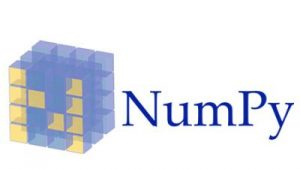NumPy is a core library for scientific computing in Python. In this cheat sheet from DataCamp you will find cheat codes for creating NumPy arrays, performing mathematics operation on array, subsetting, slicing, indexing & array manipulation. The unique aspect of this cheat sheet is it gives each function has been categorized & explained in simple English.

### 4. Exploratory Data Analysis in Python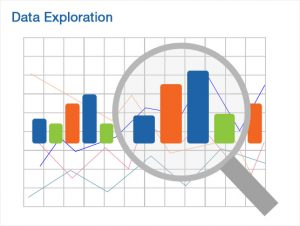Your best resource to perform data exploration in Python using NumPy, Pandas & Matplotlib. With this cheat sheet you will learn how to load files in python, convert variables, sort data, create plots, create sample datasets, treat missing values & many more. It is one of the simplified cheat sheet on data exploration.

### 5. Data Exploration using Pandas in Python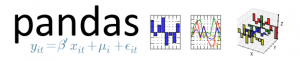Pandas is one of the important libraries in Python. This cheat sheet on data exploration operation in Python using Pandas is your go-to resource to know each step involved in data exploration. You will find cheat codes for reading & writing data, preview of dataframes, rename columns of dataframe, aggregate the data, etc.

### 6. Data Visualisation in Python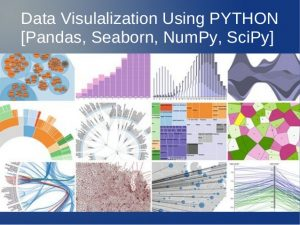Be it a data scientist or a non-techie, visualization is easily interpreted by both. In visual graphs & plots, data comes to life & speaks for itself. In this cheat sheet, learn how to perform data visualization in Python. Explore the different ways in which you can plot your data. Find step by step approach to plot histograms, bar charts, line graph, scatter plot, etc.

### 7. Python For Data Science Cheat Sheet Bokeh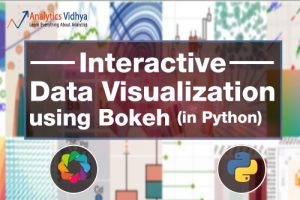This cheat sheet on Bokeh, an interactive visualization library in Python is especially useful with large datasets. In this cheat sheet by DataCamp, you will get basic steps for plotting, renderers & visual customization, save plots & create statistical charts.

### 8. Cheat Sheet: Scikit Learn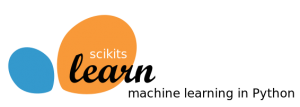Here is a cheat sheet on scikit-learn for each technique in Python. It provides different functions used for pre-processing, regression, classification, clustering, dimensionality reduction, model selection & metric along with their description. The unique aspect of this cheat sheet is it depicts the complete stages of machine learning.

### 9. Steps To Perform Text Data Cleaning in PythonText cleaning can be a cumbersome process. And knowing the right procedures is the key to getting the desired result. Refer this cheat sheet to perform text data cleaning in Python step by step. Follow this cheat sheet to know when you remove stop words, punctuation, expressions, etc. The unique aspect of this cheat sheet is each step has been explained with codes & examples.

## R for Data Science Cheat Sheets

### 1.R Reference CardUse this reference sheet for cheats codes for all functions & operators under R. Understand what the different terms mean under R. It explains all the functions under data creation, data processing, data manipulation, model function, selection and many more.

### 2. Data Import in R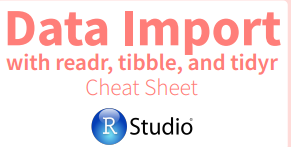Learn how to import data with readr, tibble and tidyr. Find functions to write & read functions in tibble. It also provides you useful arguments, reshape data, combine cells with tidyr.

### 3. Data Transformation with dplyr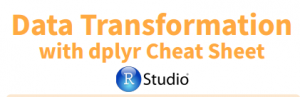This cheat sheet from RStudio is a reference material for data transformation with dplyr. Get short codes & operators for all operations under data transformation. Then be it summarize cases, group case, manipulation, vectorize & combine variables.

### 4. Cheat sheet – 11 Steps for Data Exploration in R (with codes)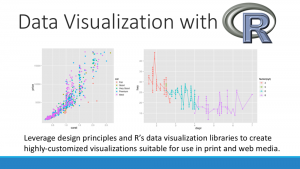This cheat sheet gives a step by step guide to  data exploration in R. Learn how to load file in R, convert variables to different data types, transpose a dataset, sort dataframe, create plots & many more.

### 5. Data Visualization in R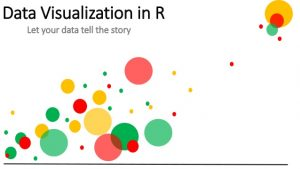Above we saw cheat sheet on data visualization in Python. Here is a data visualization cheat sheet to give the different graphs by which you can plot the data. With a few lines of code, you can create beautiful charts and data stories. R has awesome libraries to create basic and more evolved visualizations like Bar Chart, Histogram, Scatter Plot, Map visualization, Mosaic Plot and various others.

### 6. Data Visualization with ggplot2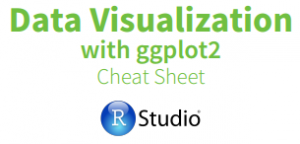This cheat sheet is specifically for creating a visualization in R using ggplot2. ggplot2 works on the grammar of graphics and is built on a set of visual marks that represent data point. Get cheat codes to create one variable & two variable graphical component. Along with different techniques for creating plots in R.

### 7. Cheat sheet: Caret Package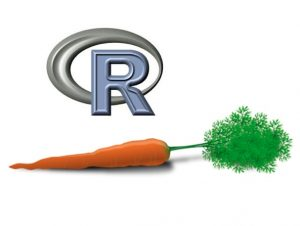Caret package provides a set of functions that streamlines the process of creating predictive models. The cheat sheet includes functions for data splitting, pre-processing, feature selection, model tuning & visualization.

### 8. R Reference Card for Data Mining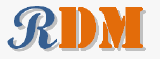This cheat sheet provides functions for text mining, outlier detection, clustering, classification, social network analysis, big data, parallel computing using R. This cheat sheet gives you all the functions & operators used for data mining in R.

### 9. Guide to quickly learn Cloud Computing in R ProgrammingCloud computing has made it very easy for us to access our files & data from anywhere. In this cheat sheet, you will learn about how to use cloud computing in R. Follow this step by step guide to use R programming on AWS.

## Machine Learning Cheat Sheets

### 1.Cheat sheet – Python & R codes for common Machine Learning Algorithms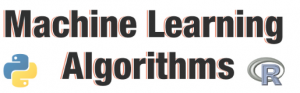In this cheat sheet, you will get codes in Python & R for various commonly used machine learning algorithms. The algorithms included are Linear regression, logistics regression, decision tree, SVM, Naive Bayes, KNN, K-means, random forest & few others.

### 2. Scikit Learn algorithm Cheat sheetThis cheat sheet is provided from the official makers of scikit-learn. Many people face the problem of choosing a particular machine learning algorithm for different data types & problems. With the help of this cheat sheet, you have the complete flow for solving a machine learning problem.

### 3. Microsoft Azure Machine Learning: Algorithm Cheat SheetThis cheat sheet helps you choose the best Azure Machine Learning Studio algorithm for your predictive analytics solution. Developed by Microsoft Azure team itself cheat sheet gives you a clear path as per the nature of the data.

## Probability Cheat Sheets

### 1. Probability Basics  Cheat Sheet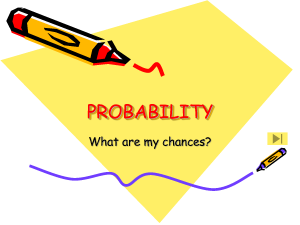This cheat sheet provides you a comprehensive reference material for probability & statistics. Each concept has been explained marvelously with a diagrammatical explanation. It covers from the basic probability rules to advanced statistical concepts in a very precise & accurate manner. Developed by the University of Pennsylvania, it is one of the most comprehensive cheat sheets you can lay your hands on.

### 2. Probability cheat sheet for distribution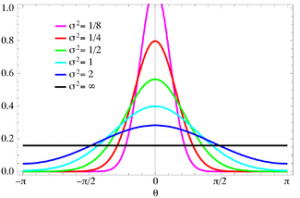Refer this cheat sheet for a quick overview on Poisson Distribution, Normal distribution, Binomial Distribution, Geometric Distribution and many more. It gives notation, formulas & a brief explanation in simple English for each distribution.

## SQL & MySQL Cheat Sheets

### 1. SQL Cheat Sheet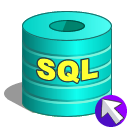In this cheat sheet, learn how to perform basic operations in SQL. Get function for inserting data, update data, deleting data, grouping data, order data, etc. If you have started using SQL this the best reference guide.

### 2. MySQL & SQL Cheat Sheet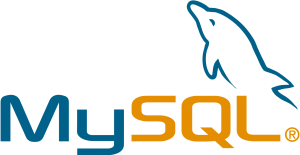In this cheat sheet, you will find commonly used MySQL & SQL commands. Get cheat codes for MySQL mathematical function, MySQL string function, basic MySQL commands. You will also find SQL commands for modifying & querying.

## Big Data Cheat Sheets

### 1. Hadoop Cheat sheet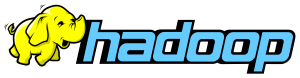It is rightly said Hadoop has a vast ecosystem & includes various operations. Learn about the various operators, how they work & what operation they are responsible for. The cheat sheet has been broken down into a respective general function like distributed systems, processing data, getting data in/out & administration.

### 2. Apache Spark Cheat sheet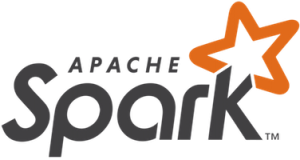Here is a cheat sheet for Apache Spark for various operations like transformation, actions, persistence methods, additional transformation & actions, extended RDD, streaming transformation, RDD persistence, etc.

### 3. Hive Function Cheat Sheet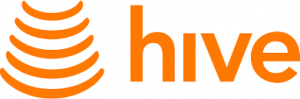In this cheat sheet, get commands for Hive functions. It provides cheat codes for data functions, mathematical function, string function, collection function, built-in aggregate function, built-in table generating function, conditional function and functions for text analytics.

### End Notes

• 144
• 0
• 819喜欢 | 0

• 0条回应给“Top 28 Cheat Sheets for Machine Learning, Data Science”的评论